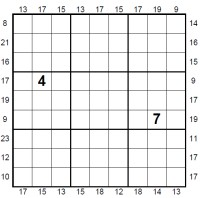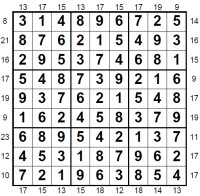Tue, 28 Jun 2022

# Outside Sudoku

Outside Sudoku Instructions:
Here's how to play Outside Sudoku:
Place the numbers from 1 - 9 once in each row, column and 3x3 box in the grid. Additionally ensure that the first three numbers in each row/column or at the end of each row/column match the listed constraint: for instance summing to the number stated at the start/end of that column.

## It's Cold Outside...

Outside sudoku puzzles are a class of puzzles that have clues outside. There are two different types of puzzles that are called outside sudoku. The first of these is a type of sudoku where numbers are presented around the edge of the grid (not necessarily at the end of every row and column) and these tell you something about the numerical properties of the three numbers closest to it in the adjacent row or column.

The second type of puzzle does not make use of the numerical values of the numbers but simply lists one or more numbers around the edge of the puzzle and this tells you that those numbers are to appear in the first three cells of that row or column. It is the first that is being discussed here.

Take a look at the sample puzzle and solution to get a clear feel for this. You will see that in this instance the numbers in the first three/last three cells of each row and column sum to the number listed at the relevant boundary of each region.

For instance, look at the first three cells of the puzzle solution image above: you'll see that they are 3, 1, 4. These sum to '8' which is the number given at the start of the row.

So this particular puzzle, sometimes called Addoku or Sudoku + in addition to outside sudoku, is a sum version of this puzzle.

Solving the outside sudoku puzzles requires then a mix of sudoku logic with that one uses when solving kakuro: the puzzle can be thought of as literally a combination of the two puzzles, or perhaps a partial killer sudoku.

Sometimes it is helpful to use the implication for the values in the middle box when solving outside sudoku, at other times you won't need that information as the puzzle won't be hard enough to require it. For instance, if the sum for the first three cells of a column is 13, and the final three cells in that column is 17, then you also know the sum for the middle sums, which is 40 - 13 - 17 = 15. Look at the three solution cells in the first column and indeed you'll see in the sample image these are 5, 9, 1 which indeed sum to 15.

It is not always the case that all sums are given around the edge of the grid, although they usually are. That said there are not too many examples of this puzzle in print: it is very rarely published!

### Related puzzles

Arrow Sudoku, Calcudoku, Circle Sudoku, Consecutive Sudoku, Futoshiki, Graeco-Latin Sudoku, HyperSudoku, Irregular Sudoku, Isosudoku, Killer Sudoku, Offset Sudoku, Samurai Sudoku, Star Sudoku, Sudoku, Sudoku X, Toroidal Sudoku

Last updated: 20 Feb 2012

Sample puzzle:Sample solution:View a sample Outside Sudoku PDF

Play Outside Sudoku Puzzles:

To play outside sudoku, then you can download and print a PDF containing 100 of these puzzles under the name of Sudoku + Magazine at our Puzzle Magazines site.

Publish Outside Sudoku Puzzles: